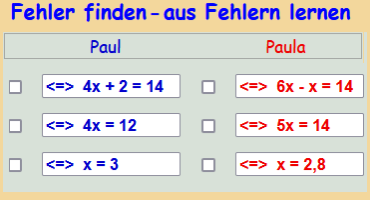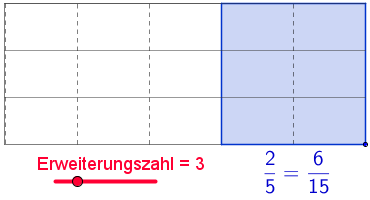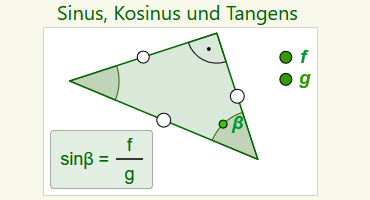## Cooperation with lehrer-online.de

Over a period of more than seven years, numerous teaching examples were developed in cooperation with Dr. Diesel from lehrer-online, which demonstrate in a special way the possibilities of interactive dynamic worksheets for teaching practice.

Lesson sequences were developed for the following topics. All examples presented are based on my own mathematics lessons and have been used by me several times in class.

The corresponding teaching unit with all freely usable materials can be found on lehrer-online.de.2005

### Rotation of vectors with GeoGebra

Through experimentation and systematic trial and error, students work out the relationship between the coordinates of the original and image vectors when they are rotated by 90° bzw. - 90°…2005

### Explore linear functions interactively

Representing facts graphically and being able to read and interpret facts from graphs are basic skills…2005

### Recognize mistakes - learn from mistakes

In mathematics lessons, sustainable learning success can be achieved if you learn to recognize errors, describe their causes and implement knowledge about errors productively…2005

### Expanding Fractions

Dynamic worksheets give students an experimental, interactive, and novel approach to understanding that the same number corresponds to different fractions of equal value.…2006

### Addition of integers - dynamically discovered

Finding the calculation rules of addition of integers in this way independently becomes an interesting and exciting journey of discovery into the world of negative numbers…2006

### Subtraction of integers with GeoGebra

((-4)) - ((-5)) = +1 is one of the problems that need to be explained with vivid support when introducing subtraction of whole numbers to students. With illustration for understanding…2006

### Minor and vertex angles - deepening with GeoGebra

The relationships between minor and vertex angles are good tools for promoting mathematical reasoning. To discover the relationship between these angles on your own…2007

### Slope of a straight line - develop with GeoGebra

The slope of a straight line is one of the central principles for understanding linear functions. An approach based on the experiences of the students…2007

### Trigonometry with GeoGebra - a variable exercise concept

With conventional teaching materials it is difficult to develop a differentiated practice environment for sine, cosine and tangent in a right triangle, which the current knowledge…